Next: Green's Theorem Up: Electrostatic Fields Previous: Electric Dipoles

# Charge Sheets and Dipole Sheets

The electric potential due to a charge sheet (i.e., a charge distribution that is confined to a surface) can be obtained from Equation (162) by replacing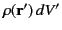with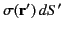. Here,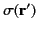is the surface charge density (i.e., the charge per unit area) at position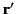. We obtain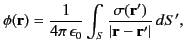(202)

where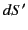is an element of the surface, on which the charges are distributed, located at position vector. Incidentally, we are assuming that the distribution is negligibly thin in the direction normal to the surface. As is well known, application of Gauss' law to a thin pill-box aligned with an element oftells us that there is a discontinuity in the normal electric field across the sheet. In fact,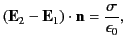(203)

where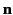is a unit normal at a given point on the sheet,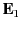and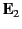are the electric fields immediately to either side of the sheet at this point (being the field on the side toward whichis directed), andis the local charge density. As is also well known, integration of Equation (156) around a small loop that straddles the sheet reveals that there is no discontinuity in the tangential electric field across the sheet.

The electric potential due to a dipole sheet (i.e., a dipole distribution that is confined to a surface) can be obtained from Equation (201) by replacing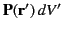with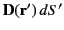. Here,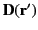is the surface dipole density (i.e., the dipole moment per unit area) at position. We obtain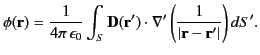(204)

We are again assuming that the distribution is negligibly thin in the direction normal to the surfaceon which the dipoles are distributed. Suppose that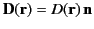, whereis a unit normal to the sheet at position vector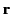. In other words, suppose that the constituent dipoles are all locally perpendicular to. It follows that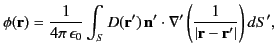(205)

where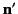is the unit normal toat position. Now, according to Equation (154) and (202), the normal electric field generated by a charge sheet is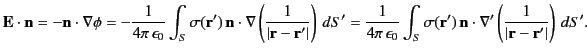(206)

A comparison between Equations (203), (205), and (206) reveals that there is a discontinuity of the electric potential across a dipole sheet. In fact,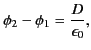(207)

where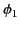and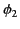are the potentials immediately to either side of a given point on the sheet [being the potential on the side toward which(and, hence,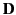) is directed], and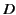is the magnitude of the local dipole density. [We can neglect the distinction betweenin Equation (205), andin Equation (206), because the discontinuous part of the electric field due to a current sheet (as well as the discontinuous part of the potential due to a dipole sheet) is generated locally.] Incidentally, there is no discontinuity in the normal electric field across a dipole sheet because the local charge density is zero. Hence, although the potential is discontinuous across a dipole sheet, the normal derivative of the potential is continuous. Likewise, there is no discontinuity in the electric potential across a charge sheet because the local dipole density is zero. Thus, although the normal derivative of the potential is discontinuous across a charge sheet (because the normal electric field is discontinuous), the potential itself is continuous.

As an example, consider a charge sheet of uniform charge densitythat corresponds to the plane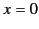. Gauss' law, in combination with symmetry arguments, reveals that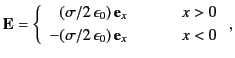(208)

which is in accordance with Equation (203). It follows from Equation (154), and the requirement that the electric potential be continuous across the sheet, that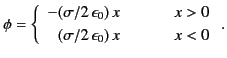(209)

Consider, now, a dipole sheet of uniform dipole density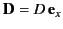that corresponds to the plane. We can think of this sheet as a combination of two charge sheets: the first, of charge density, located at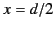, and the second, of charge density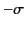, located at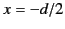. In the limit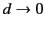, but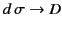, the two charge sheets are equivalent to the dipole sheet. It follows, from the previous two equations, that the electric field, and potential, generated by the dipole sheet are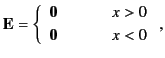(210)

and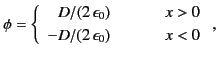(211)

respectively. The latter equation is in accordance with Equation (207). Note that, although the dipole sheet does not generated an external electric field, its internal field accelerates any charge that crosses the sheet. In fact, assuming that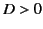, a positive charge gains energy by crossing the sheet from the region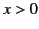to the region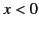.

As a second example, consider a charge sheet of uniform charge densitythat lies on the surface of a sphere, of radius, centered on the origin. Gauss' law, in combination with symmetry arguments, reveals that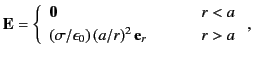(212)

where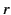is a spherical polar coordinate. The above expression is again in accordance with Equation (203). It follows from Equation (154), and the requirement that the electric potential be continuous across the sheet (as well as zero at infinity), that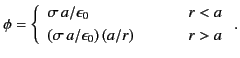(213)

Consider, now, a dipole sheet of uniform dipole density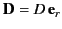that lies on the surface of the sphere. We can think of this sheet as a combination of two charge sheets: the first, of charge density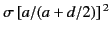, located at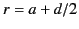, and the second, of charge density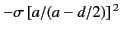, located at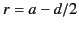. (The factors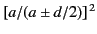are needed to ensure that both sheets contain equal and opposite net charge.) In the limit, but, the two charge sheets are equivalent to the dipole sheet. It follows, from the previous two equations, that the electric field, and potential, generated by the dipole sheet are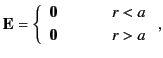(214)

and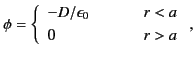(215)

respectively. The latter equation is in accordance with Equation (207). As before, the dipole sheet does not generate an external electric field, but its internal field is capable of accelerating a charge that crosses the sheet.Next: Green's Theorem Up: Electrostatic Fields Previous: Electric Dipoles
Richard Fitzpatrick 2014-06-27